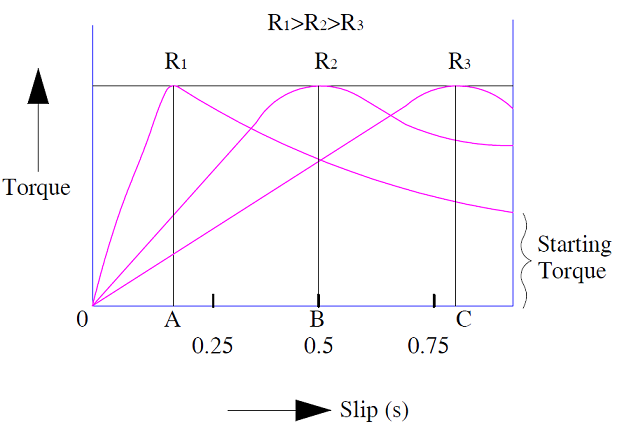# Rotor resistance Control of Induction Motor

Rotor resistance control is one among the various methods for the speed control of induction motor. In this method of speed control, the rotor circuit resistance is varied by connecting a variable external resistance. This method is only applicable for slip ring or wound rotor induction motor (WRIM). As in squirrel cage induction motor (SCIM), rotor windings terminals are not available for external connection; its speed cannot be regulated by rotor resistance control. Therefore, this method is not applicable for squirrel cage induction motor.

### Principle of Rotor Resistance Control:

We know that, the torque in an induction motor is depends on the rotor circuit resistance. Also, the maximum torque is independent of rotor resistance but the slip at which this maximum torque occurs is directly proportional to the rotor circuit resistance.

Therefore if we change the rotor resistance, the maximum torque will remain constant but the slip will increase. Figure below shows the torque slip characteristics for three different rotor resistance R1, R2 and R3.In the above figure, the maximum torque is same for rotor resistance R1, R2 and R3 but the slip increase from point A to B & C. This means, increasing rotor resistance results in increase in slip. Increase in slip in turn means reduction in induction motor speed. Thus we can say that by rotor resistance control, we can achieve variable speed at a constant torque. This is the reason; this method is suitable for constant torque drive.

It may also be noted from the above torque slip characteristics that, starting torque increases with increase in rotor resistance. Therefore this method is advantageous where we require high starting torque.

In spite of the above two advantages of rotor resistance control, this method have some disadvantage:

• This method cannot be employed for speed control of squirrel cage induction motor. This is because of non-availability of rotor winding terminals for external resistance connection.
• This method is not very efficient. Losses in external resistance and losses in carbon brushes at high slip operation cause wastage of energy.

This site uses Akismet to reduce spam. Learn how your comment data is processed.